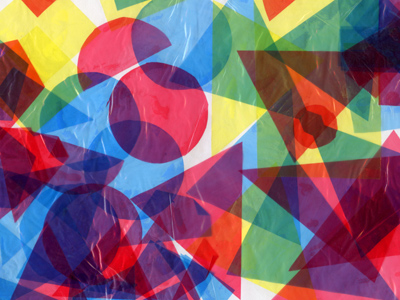See if you can get full marks in this maths quiz.

# Level 3-4 Shapes - Getting Started

The part of the KS3 Maths syllabus that is commonly called 'Shapes' also deals with measurements. This quiz introduces you to some of the words you will need for this section. It will help in getting you started in the world of shapes.

Shapes can be 2 or 3 dimensional. Rectangles and squares are 2D shapes. We call them polygons and, because their angles are the same at each corner, we call them regular polygons. Cuboids, pyramids and cubes are 3D shapes, or polyhedrons. There are many words used to describe polygons and polyhedrons, such as angles, area, faces, radius and circumference. But what do these words mean? You will have learned about them in your classes; now test how much you have remembered.

Read each of the following questions carefully. Make sure that you understand them before choosing your answers. Good luck!

1.
Which of the following might you find on a 'timetable'?
Arrival and departure times of buses
Number of cows in a field
Number of times that the telephone rings
Shopping days until Christmas
Timetables list events (such as train arrivals or school lessons) together with the time they will happen
2.
What is the meaning of the word 'capacity'?
The dimensions of an object
The weight of an object
The position of an object relative to the ground
The amount of space inside a hollow 3-dimensional shape
The capacity of a drinking glass is the amount of water it can hold when it is absolutely full
3.
Why would you never talk about the capacity of a square?
Because only round objects have a capacity
Because squares are 2-dimensional objects
Because squares are too difficult to measure
Because squares are too small to have a capacity
2-dimensional (sometimes written as 2-D) objects are flat and therefore they don't have a capacity
4.
What word is commonly used for mass?
Dimension
Height
Length
Weight
'Mass' and 'weight' are often used interchangeably in maths questions
5.
Two lines are ....... if they're at right angles to each other.
perpendicular
intersecting
parallel
cylindrical
The walls of a house are perpendicular to its floor
6.
The 'perimeter' is the distance around the outside of a 2-D object. What might this be called in the case of a circle?
Circumference
Curcumference
Sircumference
Surcumference
The formula to calculate the circumference of a circle is π radius2
7.
The surface enclosed within a 2-dimensional shape such as a square or a circle is called its what?
Area
Arena
Circumference
Volume
To calculate the area of a rectangle, multiply its length by its width
8.
Which of these cannot be measured against a continuous scale?
The mass of a bag of potatoes
The speed of a car
The population of a town
The temperature of a cup of tea
People are counted in whole numbers only. You can't have fractions of people
9.
Two lines that cross each other are said to what?
Intersperse
Interact
Intersect
Interrupt
Americans call crossroads intersections. That's because at a crossroads two roads cross each other and so intersect
10.
Two lines that are equidistant and not intersecting are known as what type of lines?
Geometric
Parallel
Simultaneous
Straight
Parallel lines never 'converge'